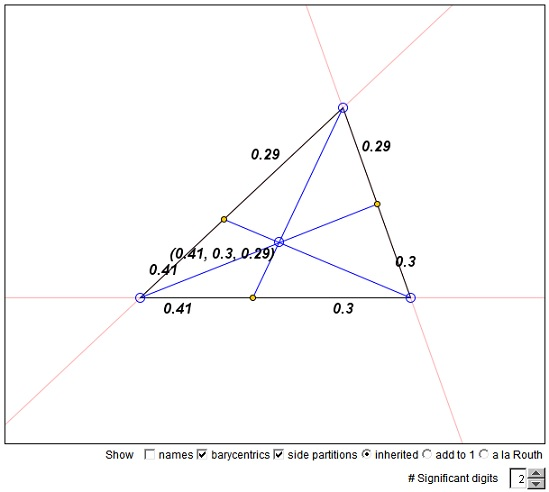# Barycentric Coordinates: A Tool

Assume that relative to ΔABC, a given point P has barycentric coordinates (α, b, γ). The cevians AP, BP, CP crosses BC, AC and AB in A', B' and C', respectively. From Ceva's theorem the product of the ratios AC'/C'B · BA'/A'C · CB'/B'A = 1. These ratios are bound to be related to the barycentric coordinates of P, but how? And the other way round: given the three ratios, do they determine the coordinates α, b, γ of P? The answer is, Yes, they do; we'll see below how.

By definition, α, b, γ can be treated as weights placed at the vertices A, B, C so that P becomes the center of gravity of the three material points:

 (1) P = (αA + bB + γC) / (α + b + γ).

The formula simpifies if we assume

 (2) α + b + γ = 1.

Rewrite (1) as

(3)
 P = αA + bB + γC = αA + (b + γ)[(b/(b + γ)·B + γ/(b + γ)·C]

Which tells us that the point K = b/(b + γ)·B + γ/(b + γ)·C lies on BC whereas P belongs to AK. In our notations, K = A' and

 (b + γ)·A' = bB + γC.

Or

 b(A' - B) = γ(C - A').

It follows that

 (4) BA' / A'C = γ / β.

A', therefore, is the center of gravity of the material points B and C with weights β and γ.

The identity (3) provides a step-by-step construction of the barycenter of three material points. First, find the barycenter of any two points and place there their combined weight. Next, find the barycenter of the so obtained and the remaining third point.

(4) answers one question: the cevians through point P with the barycentric coordinates (α, β, γ) cut the sides BC, AC, and AB in the ratios

 (5) BA' / A'C = γ / β, CB' / B'A = α / γ, AC' / C'B = β / α.

Conversely, assume that the points A', B', C' are given so that

 (6) BA' / A'C = λ, CB' / B'A = μ, AC' / C'B = ν,

with λμν = 1. What are the barycentric coordinates α, β, γ of point P whose existence is implied by Ceva's theorem?

We are looking for α, β, γ, which from (5) and (6) satisfy

 (7) γ / β = λ, α / γ = μ, β / α = ν.

Recollect that the barycentric coordinates are homogeneous and in the absence of additional restrictions are not unique. With this in mind, set in the first equation in (7) β = 1. This forces γ = λ. From the second equation then we get α = γμ = λμ. Finally, from the third equation β = λμν, which we observe does not contradict β = 1 because of the Ceva's condition λμν = 1.

P therefore has the coordinates

 (8) P = (λμ, 1, λ), and equally well, P = (μ, μν, 1), or P = (1, ν, λν).

Note that these are exactly the expressions that appear in Routh's theorem, except that now the three are equal due to the Ceva's condition λμν = 1.

The applet below is a simple tool that demonstrates the connection of the barycentric coordinates of pPoint P relative to ΔABC and the ratios in which the cevians through P split the side of the triangle. The applet represents three of the many ways in which these ratios can be written.

### If you are reading this, your browser is not set to run Java applets. Try IE11 or Safari and declare the site https://www.cut-the-knot.org as trusted in the Java setup.What if applet does not run?### Barycenter and Barycentric Coordinates